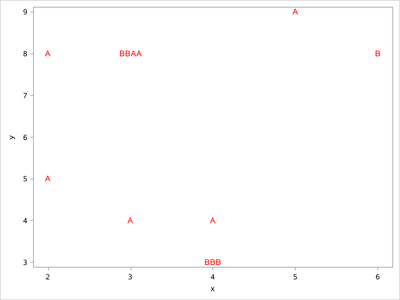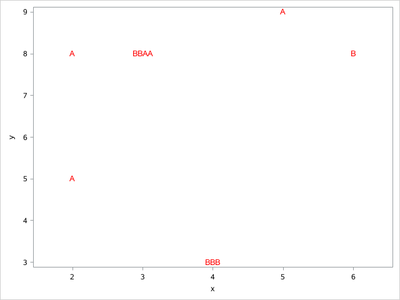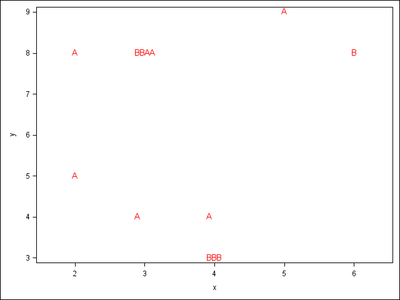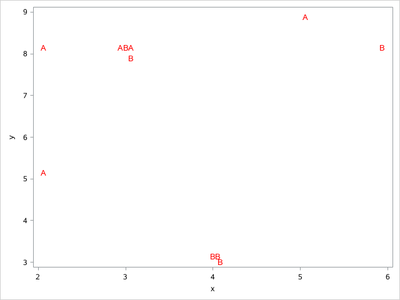## PROC SGPLOT use JITTER option with MARKERCHAR option

Hi,

I am currently have code to plot data points that are visually represented based on the value of the "Outlier" variable using the MARKERCHAR option, which has values "A" or "B" (points show up as A or B on the plot). I have recently run into a situation where there are multiple points that have the same values, and they only show up as one point. I tried using the JITTER option, but it does not seem to work with MARKERCHAR. If I remove the MARKERCHAR option, JITTER works fine, and the multiple points are shown. Is there any workaround to have both options work? My code is below. Thanks!

``````PROC SGPLOT DATA = Data_Outliers  DESCRIPTION = "Scatter Box Plot" NOAUTOLEGEND ;
VBOX Value / CATEGORY=An_Run MEANATTRS=(SIZE=0) NOOUTLIERS FILLATTRS=(COLOR="Gray") PERCENTILE=4 ;
SCATTER X = An_Run Y = Anal_Biol / JITTER MARKERCHAR=Outlier MARKERCHARATTRS=(COLOR="Red") ;
RUN ;``````

1 ACCEPTED SOLUTION

Accepted SolutionsKsharp
Super User

## Re: PROC SGPLOT use JITTER option with MARKERCHAR option

``````/*
You could combine them together
*/
data have;
input x y outlier \$1.;
cards;
2 5 A
3 8 B
3 8 B
3 8 A
3 4 A
3 8 A
3 9 B
4 3 B
4 3 B
4 3 B
4 4 A
6 8 B
5 9 A
2 8 A
;

proc sort data=have out=temp;by x y;run;
data want;
do until(last.y);
set temp;
by x y;
length label \$ 80;
label=cats(label,outlier);
end;
run;
proc sgplot data=want noautolegend;
scatter x=x y=y /markerchar=label markercharattrs=(color=red size=10) labelstrip ;
run;``````7 REPLIES 7

## Re: PROC SGPLOT use JITTER option with MARKERCHAR option

Hello @aabdesse ,

If jittering is requested with Marker Characters at a high DPI, then the marker characters seem to collide.

It's not considered as a bug.

I think it's in the documentation somewhere ...
... that jittering is not supported when MARKERCHARACTER= or MARKERSIZERESPONSE= is in effect.

SAS does not always issue a LOG message when interaction of options like this happens.

So, the behaviour you see is documented.
However , how to achieve what you want to achieve? About the latter, I still need to think a bit. 😉

More news later (hopefully).

Cheers,
KoenKsharp
Super User

## Re: PROC SGPLOT use JITTER option with MARKERCHAR option

``````/*
you should post some data to test your code.
*/
data have;
input x y outlier \$;
cards;
2 5 A
3 8 B
3 8 B
3 8 A
3 8 A
4 3 B
4 3 B
4 3 B
6 8 B
5 9 A
2 8 A
;

proc sort data=have out=temp;by x y;run;
data temp;
set temp;
by x y;
if first.y then group=0;
group+1;
run;
proc sgplot data=temp noautolegend;
scatter x=x y=y /markerchar=outlier markercharattrs=(color=red size=10)
group=group groupdisplay=cluster clusterwidth=0.3;
run;``````## Re: PROC SGPLOT use JITTER option with MARKERCHAR option

This looks good! There is only one issue I encounter when doing it this way. If I add more y values for the same x values (on the same vertical line), then the values that are not grouped together are not centered with the vertical line corresponding to the x value. I've added a few values to your example to illustrate it. Is there any way to keep everything centered? With my actual dataset, I also have boxplots in the same plot, and it looks odd when the points are not centered. Thanks!

``````data have;
input x y outlier \$;
cards;
2 5 A
3 8 B
3 8 B
3 8 A
3 4 A
3 8 A
4 3 B
4 3 B
4 3 B
4 4 A
6 8 B
5 9 A
2 8 A
;

proc sort data=have out=temp;by x y;run;
data temp;
set temp;
by x y;
if first.y then group=0;
group+1;
run;
proc sgplot data=temp noautolegend;
scatter x=x y=y /markerchar=outlier markercharattrs=(color=red size=10)
group=group groupdisplay=cluster clusterwidth=0.3;
run;``````Ksharp
Super User

## Re: PROC SGPLOT use JITTER option with MARKERCHAR option

``````/*
This code is more simple.
DATALABEL has the same effect with JITTER.
*/

data have;
input x y outlier \$;
cards;
2 5 A
3 8 B
3 8 B
3 8 A
3 8 A
4 3 B
4 3 B
4 3 B
6 8 B
5 9 A
2 8 A
;

proc sgplot data=have noautolegend;
scatter x=x y=y /datalabel=outlier markerattrs=(size=0) datalabelattrs=(size=10 color=red) ;
run;``````## Re: PROC SGPLOT use JITTER option with MARKERCHAR option

The code above is indeed simpler, however it offsets the position on the y axis when there are more than 2 values, whereas your previous example keeps them all on the same level (which for me is important as I am dealing with continuous values on the y-axis as opposed to discrete ones like in your example).Ksharp
Super User

## Re: PROC SGPLOT use JITTER option with MARKERCHAR option

``````/*
You could combine them together
*/
data have;
input x y outlier \$1.;
cards;
2 5 A
3 8 B
3 8 B
3 8 A
3 4 A
3 8 A
3 9 B
4 3 B
4 3 B
4 3 B
4 4 A
6 8 B
5 9 A
2 8 A
;

proc sort data=have out=temp;by x y;run;
data want;
do until(last.y);
set temp;
by x y;
length label \$ 80;
label=cats(label,outlier);
end;
run;
proc sgplot data=want noautolegend;
scatter x=x y=y /markerchar=label markercharattrs=(color=red size=10) labelstrip ;
run;``````## Re: PROC SGPLOT use JITTER option with MARKERCHAR option

That's perfect! Thank you so much!

Discussion stats
• 7 replies
• 553 views
• 6 likes
• 3 in conversation